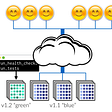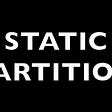# More patterns for coding interviews ||

Unpopular patterns that show up often in coding interviews

## 4- Multisource BFS

This pattern is used when you need to traverse all points at a similar depth level at once and process them according to the problem statement or find the shortest distance starting from multiple points

It's very similar to ordinary BFS except you pop the whole queue each step instead of poping one node only

Examples:

1162. As Far from Land as Possible

`class Solution:    def maxDistance(self, grid: List[List[int]]) -> int:        n, level = len(grid), 0        queue = deque([(i, j) for i, j in product(range(n), range(n)) if grid[i][j]])        if len(queue) in (0,n*n): return -1        while queue:            for _ in range(len(queue)):                i,j = queue.popleft()                for x, y in [(1,0), (-1, 0), (0, 1), (0, -1)]:                    xi, yj = x+i, y+j                    if 0 <= xi < n and 0 <= yj < n and grid[xi][yj] == 0:                        queue.append((xi, yj))                        grid[xi][yj] = 1            level += 1        return level-1`

542. 01 Matrix

`class Solution:    def updateMatrix(self, matrix: List[List[int]]) -> List[List[int]]:        n, m, level, seen = len(matrix),len(matrix), 0, set()        queue = deque([(i, j) for i, j in product(range(n), range(m)) if not matrix[i][j]])                while queue:            for _ in range(len(queue)):                i,j = queue.popleft()                if (i, j) not in seen:                    seen.add((i, j))                    matrix[i][j] = level                    for x, y in [(1,0), (-1, 0), (0, 1), (0, -1)]:                        xi, yj = x+i, y+j                        if 0 <= xi < n and 0 <= yj < m: queue.append((xi, yj))            level += 1        return matrix`

## 5- Running sum

This pattern solves problems where mathematical operations are repeated each step unnecessarily.
For example, 1+2+3 is (1+2)+3 so if you calculated 1+2 previously, you don’t need to repeat it, you can add 3 to the result instead. This reduces the complexity of each step from O(N) to O(1).

It works by keeping a running sum/count/multiplication…etc, either left to right (prefix), right to left (suffix), or both.

Examples

`class Solution:    def minFlipsMonoIncr(self, s: str) -> int:        zeros_to_left, ones_to_right, minflips = *(len(s)+1),*(len(s)+1), float('inf')                for i, x in enumerate(s): zeros_to_left[i+1]=zeros_to_left[i]+(x=='0')        for i, x in enumerate(s[::-1]): ones_to_right[-i-2]=ones_to_right[-i-1]+(x=='1')        for i in range(len(s)+1): minflips = min(minflips, len(s)-zeros_to_left[i]-ones_to_right[i])                    return minflips`

238. Product of Array Except Self

`class Solution:    def productExceptSelf(self, nums: List[int]) -> List[int]:        left_prod, right_prod = *len(nums), *len(nums)                for i in range(1,len(nums)): left_prod[i] = left_prod[i-1] * nums[i-1]        for i in range(len(nums)-2,-1,-1): right_prod[i] = right_prod[i+1]* nums[i+1]        for i in range(len(nums)): nums[i]=left_prod[i]*right_prod[i]                    return nums`

Range addition problems are problems where you add a number k to a subarray(range) for a number of updates n. for example, given array a[0,0,0,0] and updates [[2 ,[0,1]], [3 ,[1,2]]]. for the first update a =[2, 2, 0, 0], second update a = [2, 5, 0, 0]

The algorithm: for each update, k [start, end], add k to the start and subtract it from the end. then return the prefix sum for the array

There is mathematical proof of why This algorithm works you can check it here.

Examples

`class Solution:    def RangeSum(self, n, updates):        ans = *n        for update in updates:            ans[update]+=update            if update<n-1: ans[update+1]-=update        for i in range(1, n): ans[i]+=ans[i-1]        return ans`

## 7- Matrix diagonal iteration

Diagonals and counter-diagonals in matrices have special properties which are their coordinates’ subtraction and addition, respectively, are constants.

if you consider the main diagonal [a11, a22, a33, … ] m-n is always zero
The diagonal that starts from a12 is [a12, a23, a34, ..] m-n is always -1
For counter-diagonals, m+n is a constant.

You can use this constant as a key in a hash table and process the diagonal as a flat array according to the problem statement

Examples

766. Toeplitz Matrix

`class Solution:    def isToeplitzMatrix(self, matrix: List[List[int]]) -> bool:        memo = {}        for i in range(len(matrix)):            for j in range(len(matrix)):                if i-j in memo and memo[i-j] != matrix[i][j]: return False                memo[i-j] = matrix[i][j]        return True`

1329. Sort the Matrix Diagonally

`class Solution:    def diagonalSort(self, mat: List[List[int]]) -> List[List[int]]:        m, n = len(mat), len(mat)        table = {}        for i in range(n):            for j in range(m):                table[i-j] = table.get(i-j, []) + [mat[i][j]]                        for k,v in table.items(): v.sort(reverse=True)                for i in range(n):            for j in range(m):                mat[i][j] = table[i-j].pop()                 return mat`

## 8- Monotonic stack

This pattern is used to find the next greater/smaller element and the previous greater/smaller element for each element in a given sequence in linear time

let’s assume you want to find the next greater element, you would pop all elements smaller than this element, change their next greater element to be the current one, then add the current one to the stack.

with small modifications, you can find the next smaller and previous greater or smaller elements

Examples:

496. Next Greater Element I

`class Solution:    def nextGreaterElement(self, nums1: List[int], nums2: List[int]) -> List[int]:        stack, ans = [], [-1] * len(nums2)        for i, num in enumerate(nums2):            while stack and nums2[stack[-1]] < num: ans[stack.pop()] = num            stack.append(i)        return [ans[nums2.index(num)] for num in nums1]`

907. Sum of Subarray Minimums (Facebook)

`class Solution:    def sumSubarrayMins(self, arr: List[int]) -> int:        stack, ans, arr = [], 0, [float('-inf'),*arr,float('-inf')]        for i, num in enumerate(arr):            while stack and arr[stack[-1]]>num:                j = stack.pop()                ans += arr[j] * (i-j) * (j-stack[-1])            stack.append(i)        return ans%(10**9 + 7)`

That’s it for today. clap and subscribe for more patterns/

UPDATE: Part3 is now published

--

--

--

## More from Muhammed Abogazia

human

Love podcasts or audiobooks? Learn on the go with our new app.

## My Software Engineering Internship at VMware## Deploy != Release (Part 2)## DEPLOYING WORDPRESS AND MYSQL ON AWS WITH CUSTOM VPC USING TERRAFORM## Increase or Decrease the Size of Static Partition in Linux## 3 Best Eclipse IDE Books for Java Developers## Statistics on KubeCon 2018 North America and Jobs Listings## Read and learn: 5 Kotlin Articles & Redesigned website & Persistent memory series & Software…## Your guide for choosing a Content Management System (CMS) in 2019human

## Longest Common Subsequence## How to perform range addition in O(n) time and O(1) space complexity?## I’ve Failed Most Coding Interviews, and Still Agree with How They’re Set Up## What is a Stack?